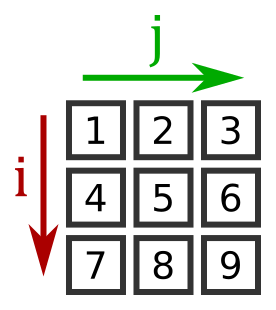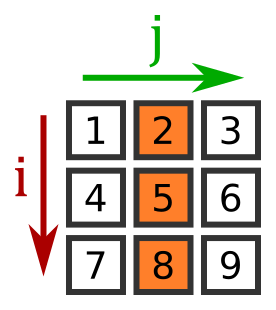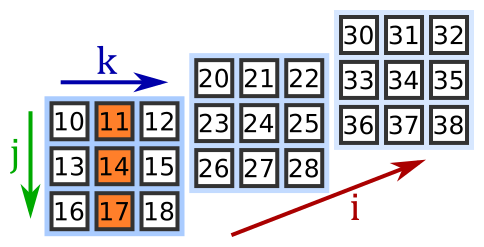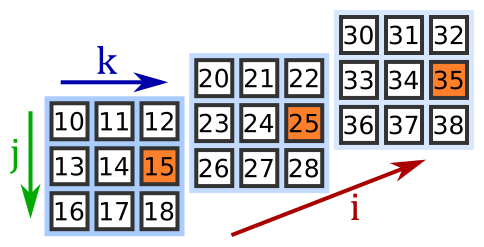# Indexing and slicing numpy arrays

Martin McBride, 2018-02-04
Tags index slice 2d arrays
Categories numpyIn this section we will look at indexing and slicing. These work in a similar way to indexing and slicing with standard Python lists, with a few differences.

Here is a video covering this topic:

## Indexing an array

Indexing is used to obtain individual elements from an array, but it can also be used to obtain entire rows, columns or planes from multi-dimensional arrays.

### Indexing in 1 dimension

We can create 1 dimensional numpy array from a list like this:

import numpy as np

a1 = np.array([1, 2, 3, 4])

print(a1)  # [1, 2, 3, 4]


We can index into this array to get an individual element, exactly the same as a normal list or tuple:

print(a1) # 1
print(a1) # 3


### Indexing in 2 dimensions

We can create a 2 dimensional numpy array from a python list of lists, like this:

import numpy as np

a2 = np.array([[1, 2, 3],
[4, 5, 6],
[7, 8, 9]])


Here is a diagram of the array:We can index an element of the array using two indices - i selects the row, and j selects the column:

print(a2[2, 1]) # 8


Notice the syntax - the i and j values are both inside the square brackets, separated by a comma (the index is actually a tuple (2, 1), but tuple packing is used). The example picks row 2, column 1, which has the value 8. This compares with the syntax you might use with a 2D list (ie a list of lists):

v = [[1, 2, 3],
[4, 5, 6],
[7, 8, 9]]

print(v) # 8


### Picking a row or column

If we can supply a single index, it will pick a row (i value) and return that as a rank 1 array:

print(a2) # [7, 8, 9]


That is quite similar to the what would happen with a 2D list. However, numpy allows us to select a single columm as well:

print(a2[:, 1]) # [2, 5, 8]


We are skipping ahead slightly to slicing, later in this tutorial, but what this syntax means is:

• for the i value, take all values (: is a full slice, from start to end)
• for the j value take 1

Giving this array [2, 5, 8]:The array you get back when you index or slice a numpy array is a view of the original array. It is the same data, just accessed in a different order. If you change the view, you will change the corresponding elements in the original array.

### Indexing in 3 dimensions

We can create a 3 dimensional numpy array from a python list of lists of lists, like this:

import numpy as np

a3 = np.array([[[10, 11, 12], [13, 14, 15], [16, 17, 18]],
[[20, 21, 22], [23, 24, 25], [26, 27, 28]],
[[30, 31, 32], [33, 34, 35], [36, 37, 38]]])


Here is a diagram of the array:A 3D array is like a stack of matrices:

• The first index, i, selects the matrix
• The second index, j, selects the row
• The third index, k, selects the column

Here is the same diagram, spread out a bit so we can see the values:Here is how to index a particular value in a 3D array:

print(a3[2, 0, 1]) # 31


This selects matrix index 2 (the final matrix), row 0, column 1, giving a value 31.

### Picking a row or column in a 3D array

You can access any row or column in a 3D array. There are 3 cases.

Case 1 - specifying the first two indices. In this case, you are choosing the i value (the matrix), and the j value (the row). This will select a specific row. In this example we are selecting row 2 from matrix 1:

print(a3[1, 2]) # [26 27 28]Case 2 - specifying the i value (the matrix), and the k value (the column), using a full slice (:) for the j value (the row). This will select a specific column. In this example we are selecting column 1 from matrix 0:

print(a3[0, :, 1]) # [11 14 17]Case 3 - specifying the j value (the row), and the k value (the column), using a full slice (:) for the i value (the matrix). This will create a row by taking the same element from each matrix. In this case we are taking row 1, column 2 from each matrix:

print(a3[:, 1, 2]) # [15, 25, 35]### Picking a matrix in a 3D array

If we only specify the i index, numpy will return the corresponding matrix. We will call this case 1. In this example we will request matrix 2:

print(a3) # [[30 31 32]
#  [33 34 35]
#  [36 37 38]]Case 2 if we specify just the j value (using a full slice for the i values), we will obtain a matrix made from the selected row taken from each plane. In this example we will take row 1:

print(a3[:, 1]) # [[13 14 15]
#  [23 24 25]
#  [33 34 35]]Case 3 if we specify just the k value (using full slices for the i and j values), we will obtain a matrix made from the selected column taken from each plane. In this example we will take column 0:

print(a3[:, :, 0]) # [[10 13 16]
#  [20 23 26]
#  [30 33 36]]## Slicing an array

You can slice a numpy array is a similar way to slicing a list - except you can do it in more than one dimension.

As with indexing, the array you get back when you index or slice a numpy array is a view of the original array. It is the same data, just accessed in a different order. This is different to lists, where a slice returns a completely new list.

### Slicing lists - a recap

Just a quick recap on how slicing works with normal Python lists. Suppose we have a list:

a = [10, 11, 12, 13, 14]


We can use slicing to take a sub-list, like this:

b = a[1:4]    # [11, 12, 13]


The slice notation specifies a start and end value [start:end] and copies the list from start up to but not including end.

We can omit the start, in which case the slice start at the beginning of the list. We can omit the end, so the slice continues to the end of the list. If we omit both the slice created is a copy of the entire list:

c = a[:3]    # [10, 11, 12]
d = a[2:]    # [12, 13, 14]
e = a[:]     # [10, 11, 12, 13, 14]


One final thing to note is the difference between an index and a slice of length 1:

f = a    # 12
g = a[2:3]  # 


The index returns an element of the array, the slice returns a list of one element.

### Slicing 1D numpy arrays

Slicing a 1D numpy array is almost exactly the same as slicing a list:

import numpy as np

a1 = np.array([1, 2, 3, 4, 5])
b = a1[1:4]

print(b)  # [2, 3, 4]


The only thing to remember if that (unlike a list) a1 and b are both looking at the same underlying data (b is a view of the data). So if you change an element in b, a1 will be affected (and vice versa):

b = 10
print(b)  # [2, 10, 4]
print(a1) # [1, 2, 10, 4, 5]


### Slicing a 2D array

You can slice a 2D array in both axes to obtain a rectangular subset of the original array. For example:

import numpy as np

a2 = np.array([[10, 11, 12, 13, 14],
[15, 16, 17, 18, 19],
[20, 21, 22, 23, 24],
[25, 26, 27, 28, 29]])

print(a2[1:,2:4])  # [[17 18]
#  [22 23]
#  [27 28]]


This selects rows 1: (1 to the end of bottom of the array) and columns 2:4 (columns 2 and 3), as shown here:### Slicing a 3D array

You can slice a 3D array in all 3 axes to obtain a cuboid subset of the original array:

import numpy as np

a3 = np.array([[[10, 11, 12], [13, 14, 15], [16, 17, 18]],
[[20, 21, 22], [23, 24, 25], [26, 27, 28]],
[[30, 31, 32], [33, 34, 35], [36, 37, 38]]])

print(a3[:2,1:,:2])  # [[ [13 14] [16 17] ]
#  [ [23 24] [26 27] ]]


This selects:

• planes :2 (the first 2 planes)
• rows 1: (the last 2 rows)
• columns :2 (the first 2 columns)

As shown here:### Full slices

You can, of course, use full slices : to select all planes, columns or rows. However, for trailing indices, simply omitting the index counts as a full slice. So for 2D arrays:

a2[1:3,:] # is the same as
a2[1:3]


For 3D arrays:

a3[1:,:2,:] # is the same as
a3[1:,:2]

a3[1:,:,:] # is the same as
a3[1:,:]   # and is also the same as
a3[1:]


### Slices vs indexing

As we saw earlier, you can use an index to select a particular plane column or row. Here we select row 1, columns 2:4:

import numpy as np

a2 = np.array([[10, 11, 12, 13, 14],
[15, 16, 17, 18, 19],
[20, 21, 22, 23, 24],
[25, 26, 27, 28, 29]])

print(a2[1,2:4])  # [17 18]


You can also use a slice of length 1 to do something similar (slice 1:2 instead of index 1):

print(a2[1:2,2:4])  # [[17 18]]


Notice the subtle difference. The first creates a 1D array, the second creates a 2D array with only one row.

If you found this article useful, you might be interested in the book NumPy Recipes or other books by the same author.

#### Popular tags

2d arrays abstract data type alignment and angle animation arc array arrays bar chart bar style behavioural pattern bezier curve built-in function callable object chain circle classes clipping close closure cmyk colour combinations comparison operator comprehension context context manager conversion count creational pattern data science data types decorator design pattern device space dictionary drawing duck typing efficiency ellipse else encryption enumerate fill filter font font style for loop function function composition function plot functools game development generativepy tutorial generator geometry gif global variable gradient greyscale higher order function hsl html image image processing imagesurface immutable object in operator index inner function input installing iter iterable iterator itertools join l system lambda function len lerp line line plot line style linear gradient linspace list list comprehension logical operator lru_cache magic method mandelbrot mandelbrot set map marker style matplotlib monad mutability named parameter numeric python numpy object open operator optimisation optional parameter or pandas partial application path pattern permutations pie chart polygon positional parameter print pure function python standard library radial gradient range recipes rectangle recursion reduce regular polygon repeat rgb rotation roundrect scaling scatter plot scipy sector segment sequence setup shape singleton slice slicing sound spirograph sprite square str stream string stroke structural pattern subpath symmetric encryption template text text metrics tinkerbell fractal transform translation transparency triangle truthy value tuple turtle unpacking user space vectorisation webserver website while loop zip